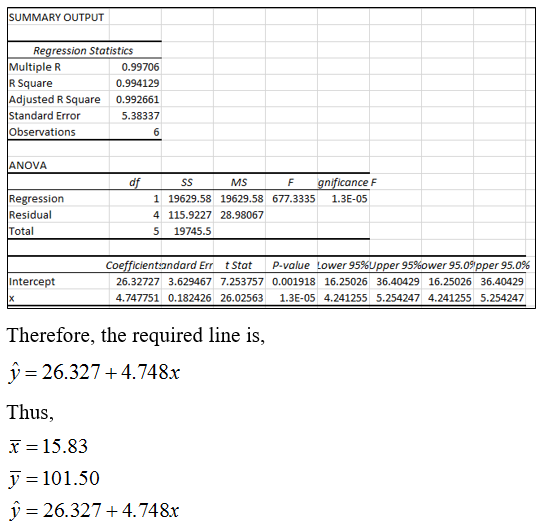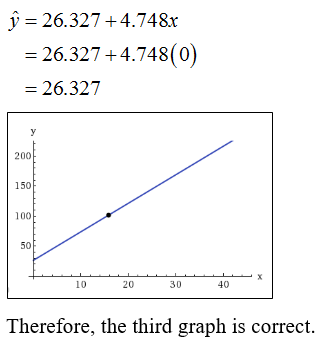Question
39 views

You are the foreman of the Bar-S cattle ranch in Colorado. A neighboring ranch has calves for sale, and you are going to buy some calves to add to the Bar-S herd. How much should a healthy calf weigh? Let x be the age of the calf (in weeks), and let y be the weight of the calf (in kilograms).

 x 1 5 11 16 26 36 y 39 47 73 100 150 200

check_circle

Step 1

Consider x and y be the age of the calf (in weeks) and the weight of the calf (in kilograms), respectively.

(c) Excel is used to obtain the required mean and least square line.

Steps for obtaining mean;

1. Enter the data into spreadsheet.
2. Use the Excel formula =Average(range of x) which gives the mean of x as 15.83.
3. Use the Excel formula =Average(range of y) which gives the mean of y as 101.50.
Step 2

Steps for obtaining least square line is,

1. Enter the data into spreadsheet.
2. Go to Data>Data analysis>Regression>ok.
3. Insert the range of Y and X and tick the labels.
4. Click ok.
5. The result is obtained as;Step 3

(d) The point of (x-mean, y-mean) is obtained as (15.83, 101.50).

The predicted value of y at x=0 is obtained as;...

### Want to see the full answer?

See Solution

#### Want to see this answer and more?

Solutions are written by subject experts who are available 24/7. Questions are typically answered within 1 hour.*

See Solution
*Response times may vary by subject and question.
Tagged in